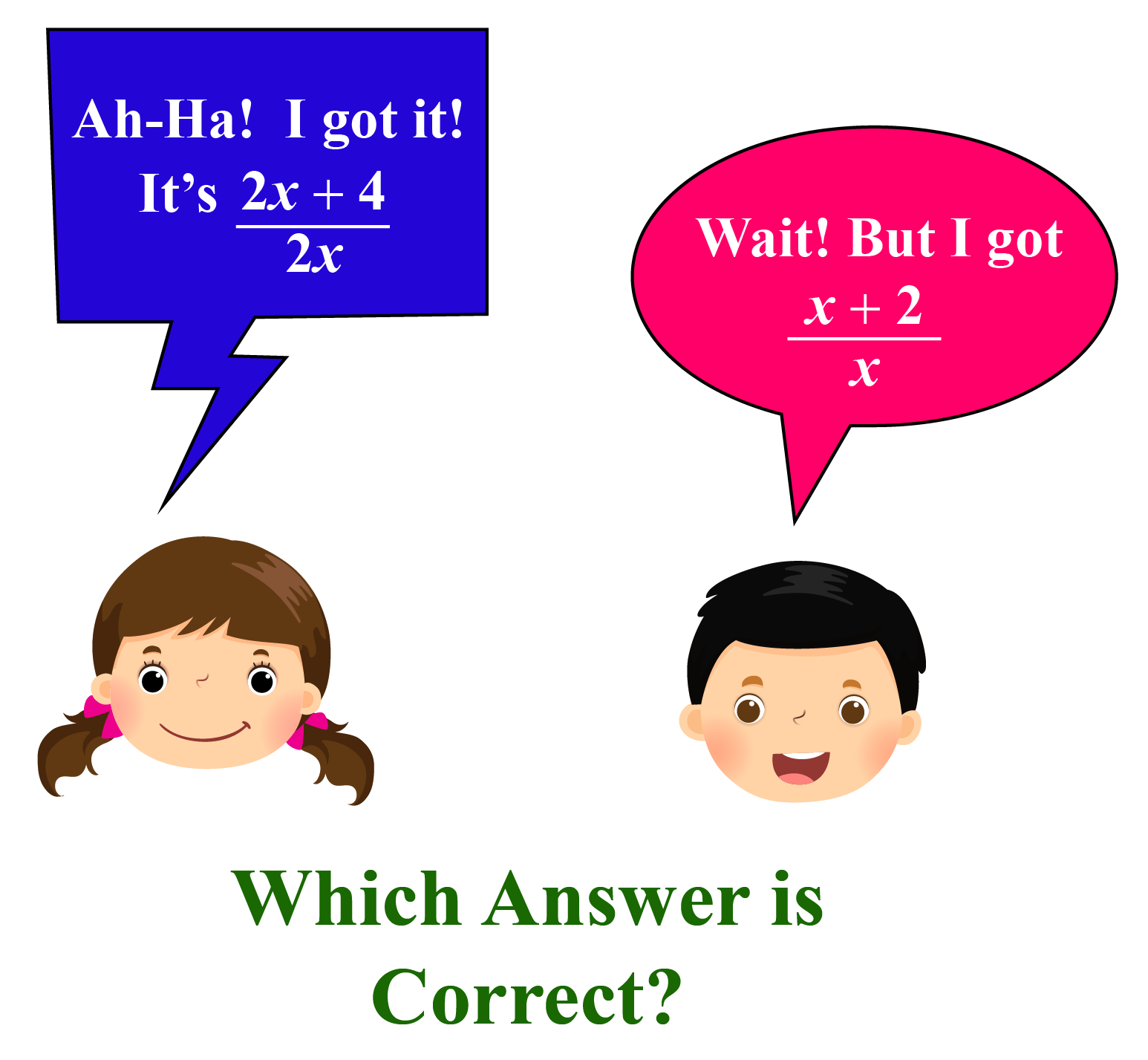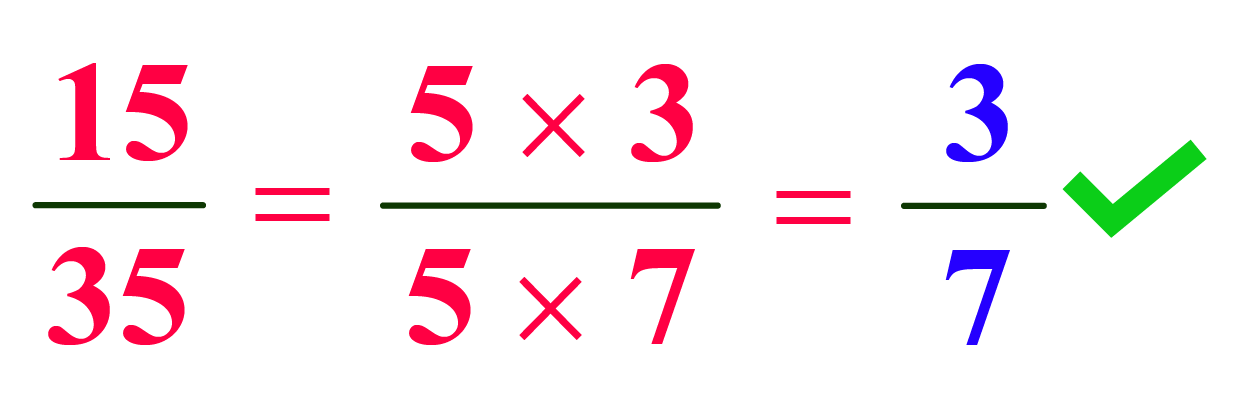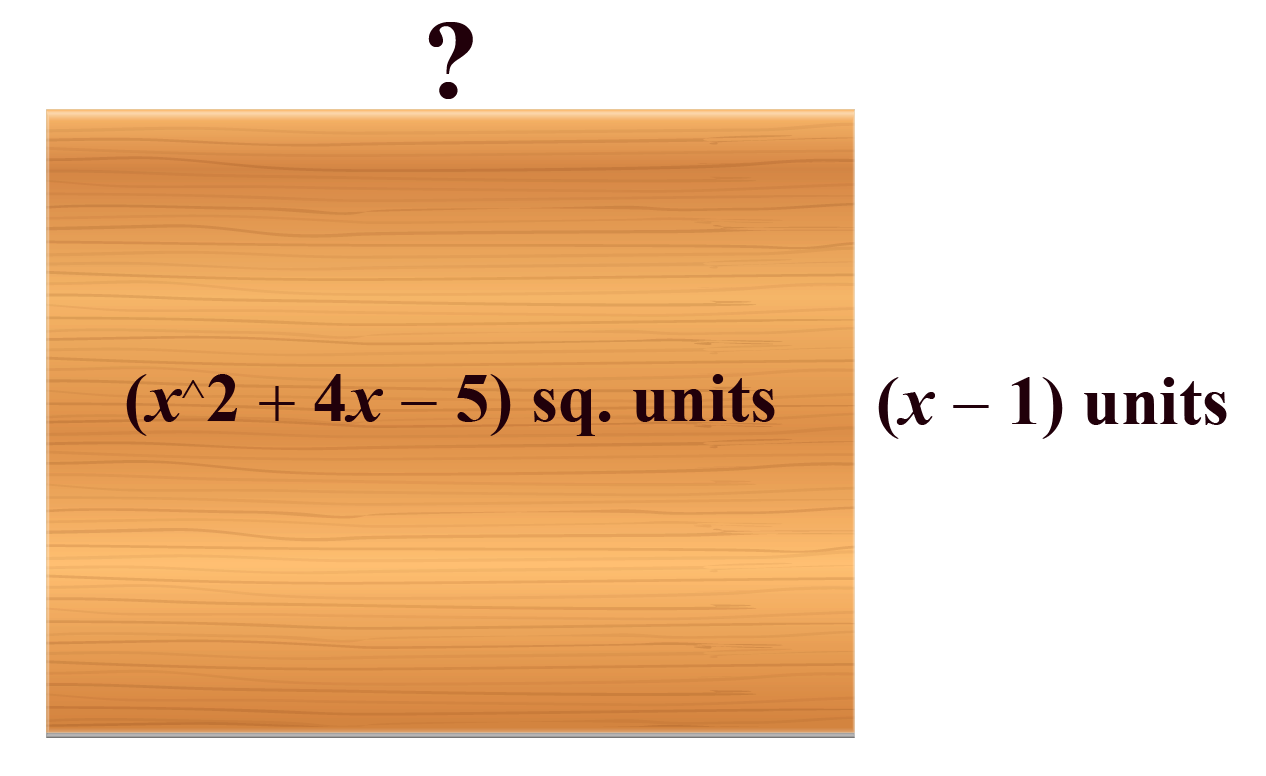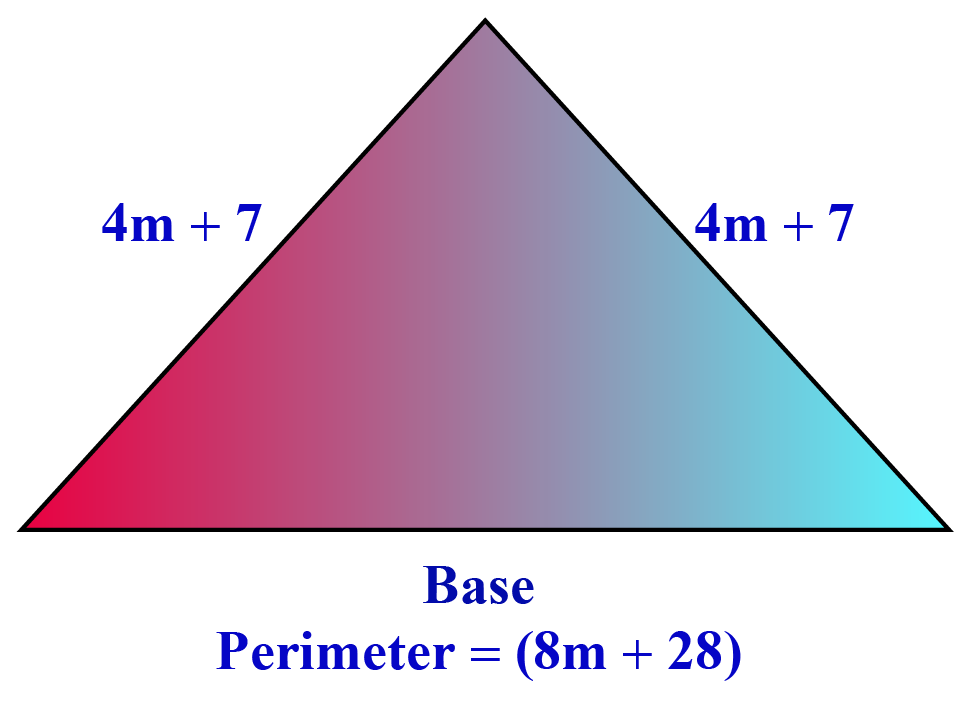# Simplifying Rational Expressions

Simplifying Rational Expressions

In this lesson, we will learn more about rational expressions by understanding how to simplify rational expressions step-by-step along with some solved examples and a few interactive questions to test your understanding.

A rational expression is a number in which both numerator and denominator are polynomials.

Simplication of a rational expression is done by converting the numerator and denominator to their lowest form.Let's move ahead and explore ways to simplify rational expressions.

## Lesson Plan

 1 What Is Meant by Simplifying Rational Expressions? 2 Important Notes on Simplifying Rational Expressions 3 Solved Examples on Simplifying Rational Expressions 4 Challenging Questions on Simplifying Rational Expressions 5 Interactive Questions on Simplifying Rational Expressions

## What Is Meant by Simplifying Rational Expressions?

Simplifying rational expressions means to reduce the value of a rational expression to its lowest form or simplified form.

When the numerator and denominator of a rational number have no common factor other than 1, we consider it in its simplified form.Note that the value of the original number and the simplified form of the same number is always the same. They are equivalent.

### Simplifying Rational Expression Calculator

In the simulation given below, write the values of numerator and denominator of a rational expression and click on SIMPLIFY to get the answer.

## How to Simplify Rational Expressions?

The simplification of a rational expression is the same as how we simplify fractions. The only difference is of having polynomials in the expression.

Let us look at the steps to be followed for simplification.

Step 1: Search for common factors in numerator and denominator.

For example: \begin{align}\frac{3y^2+6y}{6y^2+9y}\end{align} , $$y \neq\ 0$$

Factorizing the numerator and denominator, we'll get,

\begin{align}\frac{3y^2+6y}{6y^2+9y}\end{align} = \begin{align}\frac{3y(y+2)}{3y(2y+3)}\end{align}

Step 2: Cancel Common factors.

In the above expression, the common factor of numerator and denominator is 3y. So, in this step, we have to cancel that common factor.

Step 3: Write the remaining terms in the numerator and denominator.

After Cancelling common factors, we have to write down terms left with us in the numerator and denominator.

\begin{align}\frac{y+2}{2y+3}\end{align}

Step 4: Mention restricted values if any.

In this last step, we have to mention the restricted values of the given rational expression, if any. Please note, that the restricted value of the simplified expression will be the same as the restricted values of the original expression.

\begin{align}\frac{y+2}{2y+3} , y \neq 0\end{align}

Example and Solution

Let us simplify a rational expression with two variables \begin{align}\frac{x^2-xy-6y^2}{x-3y}\end{align}  to have more clarity.

Step 1: By factorizing the numerator $$x^2-xy-6y^2$$we get $$(x-3y)(x+2y)$$

$$x^2-3xy+2xy-6y^2$$

$$x(x-3y)+2y(x-3y)$$

$$(x-3y)(x+2y)$$

Step 2: Cancel $$(x-3y)$$ from both numerator and denominator.

Step 3: After cancellation, only $$x+2y$$ is left in the numerator that cannot be further reduced.

\begin{align}\therefore\ \frac{x^2-xy-6y^2}{x-3y}= x+2y\end{align}Important Notes
1. Let us revise the steps to simplify any rational expression.

Step 1: Search for common factors in numerator and denominator.

Step 2: Cancel Common factors.

Step 3: Write the remaining terms in the numerator and denominator.

Step 4:  Mention restricted values if any.

2. Original expression and the simplified expression are equivalent to each other.

Let us look at some examples of simplifying rational expressions in the next section.

## Solved Examples

 Example 1

Find the length of a rectangular tabletop whose area is given as $$(x^2+ 4x- 5)\ sq. units$$ and breadth is $$(x-1) \ units$$.Solution

We know that Length of a Rectangle=  \begin{align}\frac{Area}{Breadth}\end{align}

\begin{align}Length= \frac{x^2+4x-5}{x-1}\end{align}

\begin{align}Length= \frac{(x+5)(x-1)}{x-1}\end{align}

\begin{align}Length= (x-1)\ units\end{align}

 \begin{align}\therefore\ Length\ of\ the\ top\ is\ (x-1)\ units.\end{align}
 Example 2

The length of two equal sides of an isosceles triangle is $$4m+7$$. The perimeter of the triangle is $$8m+28$$. Determine the ratio of the perimeter to the base.Solution

The base of the triangle= Perimeter $$-$$ (Length of the other two sides)

=  \begin{align}(8m+28)- (4m+7+4m+7)\end{align}

=  \begin{align}(8m+28)- (8m+14)\end{align}

=  \begin{align}14 \ units\end{align}

The ratio of the Perimeter to Base=  \begin{align}\frac{8m+28}{14}\end{align}

=  \begin{align}\frac{2(4m+14)}{2 \times 7}\end{align}

=  \begin{align}\frac{4m+14}{7}\end{align}

 $$\therefore$$ The Ratio is \begin{align}\frac{4m+14}{7}\end{align}
 Example 3

A kid has to cover a distance of $$5y^2+25y$$ yards in $$5y$$ seconds to win the race. Find out the speed of the kid.Solution

Speed of the kid = \begin{align}\frac{Distance}{Time\ Taken}\end{align}

= \begin{align}\frac{5y^2+25y}{5y}\end{align}

= \begin{align}\frac{5y(y+5)}{5y}\end{align}

= \begin{align}(y+5) \ yards\ per\ second\end{align}

 $$\therefore$$ The speed is \begin{align} (y+5)\end{align} yards per secondChallenging Questions
1. Simplify:  \begin{align}\frac{9x^2+12x+4}{4x^2-27x-7} \div\ \frac{12x^2+5x-2}{16x^2-1}\end{align}
2. Simplify: \begin{align}\frac{x^2+10x+24}{x^2-5x+6}\end{align} $$\div$$ \begin{align}\frac{x^2+11x+28}{x^2-6x+9}\end{align} $$\times$$ \begin{align}\frac{x^2+x-42}{x^2+3x-18}\end{align}

## Interactive Questions on Simplifying Rational Expressions

Here are a few activities for you to practice. Select/Type your answer and click the "Check Answer" button to see the result.

## Let's Summarize

We hope you enjoyed learning about simplifying rational expressions with the examples and practice questions. Now, you will be able to easily solve problems on various techniques used for simplifying rational expressions and questions related to its application.

At Cuemath, our team of math experts is dedicated to making learning fun for our favorite readers, the students!

Through an interactive and engaging learning-teaching-learning approach, the teachers explore all angles of a topic.

Be it worksheets, online classes, doubt sessions, or any other form of relation, it’s the logical thinking and smart learning approach that we, at Cuemath, believe in.

## 1. How do you reduce rational expressions to the lowest terms?

Rational expressions are reduced to the lowest terms by taking out the common factor from the numerator and denominator.

## 2. How to simplify rational expressions with exponents?

To simplify rational expressions with exponents, we have to follow rules of exponents and powers and then cancel the common factor from the numerator and denominator.

## 3. How do you simplify fractional powers?

To simplify expression with fractional powers, we have to use the rules of exponents.

For example,  \begin{align}\frac{x^\frac{1}{2}}{x^\frac{1}{3}}= x^(\frac{1}{2}-\frac{1}{3})\end{align}

\begin{align}x^\frac{1}{6}\end{align} is the simplified or lowest form of   \begin{align}\frac{x^\frac{1}{2}}{x^\frac{1}{3}}\end{align}

More Important Topics
Numbers
Algebra
Geometry
Measurement
Money
Data
Trigonometry
Calculus
More Important Topics
Numbers
Algebra
Geometry
Measurement
Money
Data
Trigonometry
Calculus